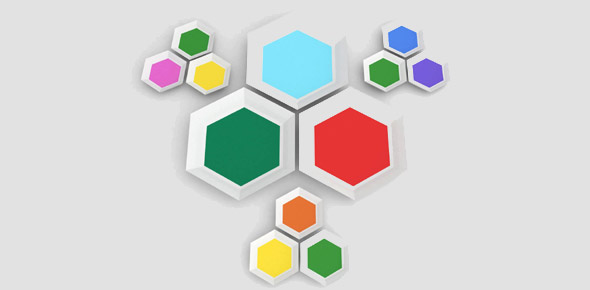# Can You Identify These Basic 3D Shapes?

10 Questions | Total Attempts: 2617SettingsA 3D shape is represented by its edges, faces, and vertices which can be measured in 3 directions known as three-dimensional shapes. The length, width, and height are the three measuring units ina 3D shape. Take this quiz to test your knowledge about different 3D shapes. So, let's try out the quiz. All the best!

Related Topics
• 1.
What shape is a 3d circle
• A.

Cube

• B.

Rectangle

• C.

Circle

• D.

Sphere

• 2.
What shape is the cross section of the sphere?
• A.

Cone

• B.

Circle

• C.

Pyramid

• 3.
What kind of shape will you get with a cross section parallel to the base
• A.

Cylinder

• B.

Circle

• C.

Cube

• 4.
Will the cross section be congruent to the base of a cylinder
• A.

No

• B.

Yes

• 5.
What is a description of a cone
• A.

A figure with a circle top

• B.

A figure with a pointed top and a circled bottom

• C.

A figure with a square top

• 6.
A 3-D shape can also be called...
• A.

A flat shape

• B.

A solid shape

• C.

A polygon

• D.

• 7.
Vertices are also called...
• A.

Edges

• B.

Corners

• C.

Faces

• D.

Sides

• 8.
A solid object has six faces which are all squares. What is the name of this object?
• A.

Cube

• B.

Cuboid

• C.

Cylinder

• D.

Rectangle

• 9.
Which 3-D shape has six rectangular faces?
• A.

Sphere

• B.

Cuboid

• C.

Cylinder

• D.

Square

• 10.
Most dice are the shape of which 3-D shape?
• A.

Cuboid

• B.

Cylinder

• C.

Cube

• D.

Square